The expressionis called a radical expression. The symbolis called the radical sign. The expression under the radical sign is called the radicand, and n, an integer greater than 1, is called the index. If the radical expression appears without an index, the index is assumed to be 2. The expressionis read as “the nth root of a.” Remember:##### Example 1

Simplify each of the following.

1.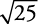2.3.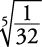4.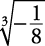5.1. If, then x 2 = 25.

x = 5 or x = –5

Because x could be either value, a rule is established. If a radical expression could have either a positive or a negative answer, then you always take the positive. This is called the “principal root.” Thus,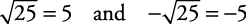2. If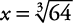, then

x 3 = 64 and  x = 43. If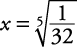, then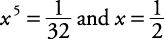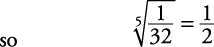4. If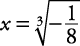, then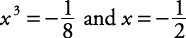5. If, then x 2 = –4. There is no real value for x, so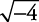is not a real number.

Following are true statements regarding radical expressions.

n

a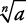Example

even

positive

negative

zero

positive

not real

zero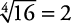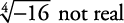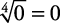odd

positive

negative

zero

negative

zero

positive

negative

zero

not real

zero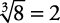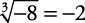When variables are involved, absolute value signs are sometimes needed.

##### Example 2

Simplify.

It would seem that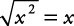. But there is no guarantee that x is nonnegative. Because of this,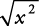is expressed as | x|, which guarantees that the result is nonnegative.

Absolute value signs are never used when the index is odd. Absolute value signs are sometimes used when the index is even, at those times when the result could possibly be negative.

##### Example 3

Simplify the following, using absolute value signs when needed.

1.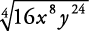2.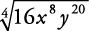3.1.Since 2 x 2 y 6 could not be negative even if x or y were negative, absolute value signs are not needed.

2.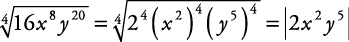Since the expression could be negative if y were negative, a correct way to represent the answer is |2 x 2 y 5|. Because only y could have caused the answer to be negative, another way to represent the answer is 2 x 2| y| 5.

3.Absolute value signs are never used when the index is odd.